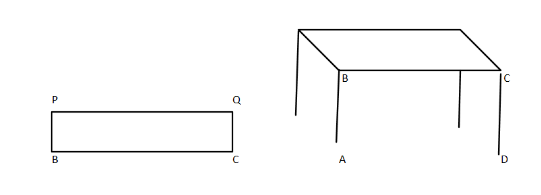A table-top measures $2m25cm$ by $1m50cm$. What is the perimeter of the table-top?Verified
141.9k+ views
Hint: You are given the length and breadth of a table and you are asked to find the perimeter of that table-top which is given by $2\left( {length + breadth} \right)$. Using this you will be able to get the answer.

Now it is given that the table-top measures $2m25cm$ by $1m50cm$. This means that the length of the table is $2m25cm$ and breadth is $1m50cm$.Here $ABCD$ is a table and $PCBQ$ is the table-top whose measurement is given by $2m25cm$ by $1m50cm$.
This means that the length of the table $PQ$$= 2m25cm and breadth BP$$ =$ $1m50cm$ and it is in the form of the rectangle.
Now the length is given in the form $x$ $m$$and y$$cm$ now we need to convert it into either $meter$ or $centimeter$. So if we try to convert $x$ $m$ $and$ $y$$cm into centimeter then it will become: \left( {\left( {x \times 100} \right) + y} \right)cm. So here PQ is given as 2m25cm, we have to change it into cm. PQ = \left( {\left( {2 \times 100} \right) + 25} \right)cm = 225cm Similarly we have to change BP$$ = $$1m50cm = \left( {\left( {1 \times 100} \right) + 50} \right)cm = 150cm Now it is asked to find out the perimeter of the table-top which is BPQC. Hence we know that BPQC is a rectangle and we know that the perimeter of the rectangle is equal to 2\left( {length + breadth} \right). Here adjacent sides are calculated as PQ$$ = 225cm$
$BP = 150cm$
Perimeter of the table top $=$ $2\left( {PQ + BP} \right)$
$= $$2\left( {225 + 150} \right)$$cm$
$=$ $2\left( {375} \right)cm$
$= 750cm$
Now we have perimeter $= 750cm$
We can write it as $= \left( {\left( {7 \times 100} \right) + 50} \right)cm$
Which means it is $7m50cm$.

Note: To convert $x$ $m$ $y$ $cm$ into $cm$, we have to use $\left( {100x + y} \right)cm$. We should know that the perimeter of the rectangle is equal to $2\left( {length + breadth} \right)$.